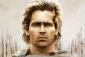# Required diameter of solid shaft

2 posts / 0 new
AlexanderRequired diameter of solid shaft

A solid shaft is to transmit 370 kW at 120 rpm. If the shearing stress of the material must not exceed 92 MPa, find the diameter required.

Tags:
jayborre

Given:

P = 370 kW = 370 x103 watts ((N-m)/s)= 3.7 〖x10〗^8 ((N-m)/s)

f = 120 rev/min x 1min/60s=2 rev/s

τ=92 N/mm^2

Solution:

1. Calculate Twisting Moment/ Torsion, T
P=2πT f

3.7 〖x10〗^8 ((N-mm)/s)=2 π T (2 rev/s)

T = 2.944〖 x 10〗^7 N-mm

2. Solve for diameter, D of solid shaft using the following formulas:
τ = Tr/J

J= π/32 D^4

r= D/2

τs= Tr/J

92 N/mm^2 = (2.944〖 x 10〗^7 N-mm (D/2))/(π/32 D)

D=117.69mm of 4.72inches

• Mathematics inside the configured delimiters is rendered by MathJax. The default math delimiters are $$...$$ and $...$ for displayed mathematics, and $...$ and $...$ for in-line mathematics.